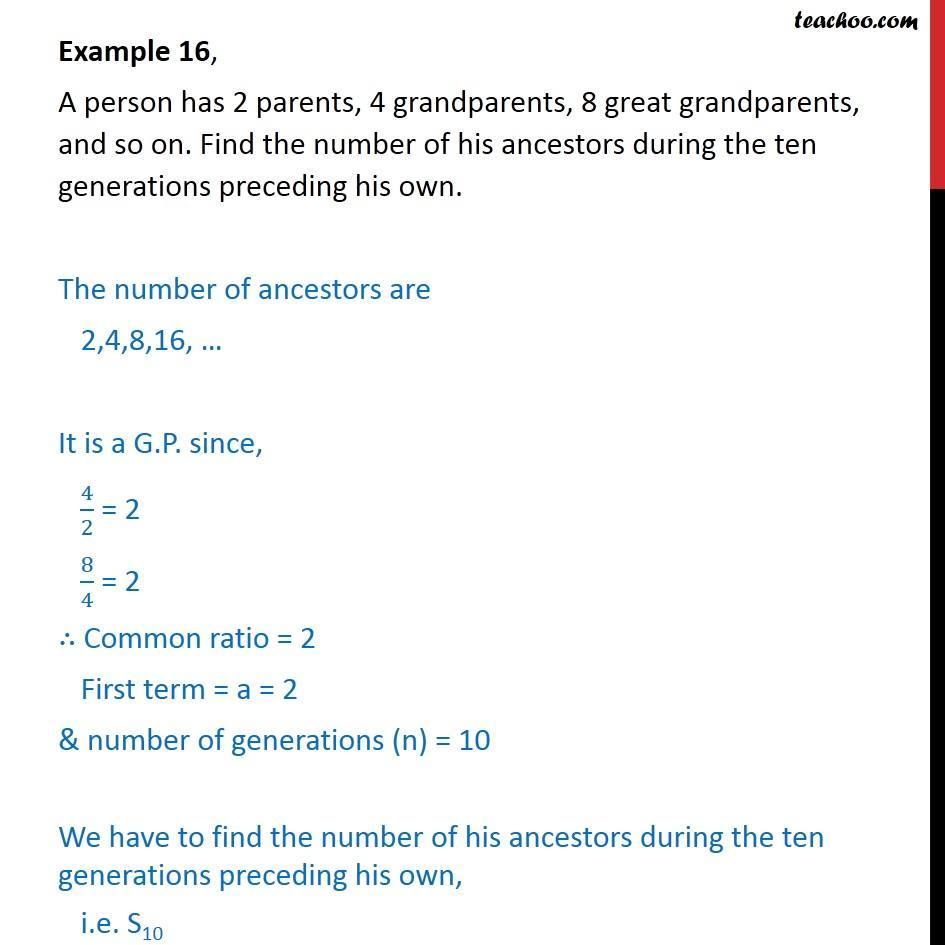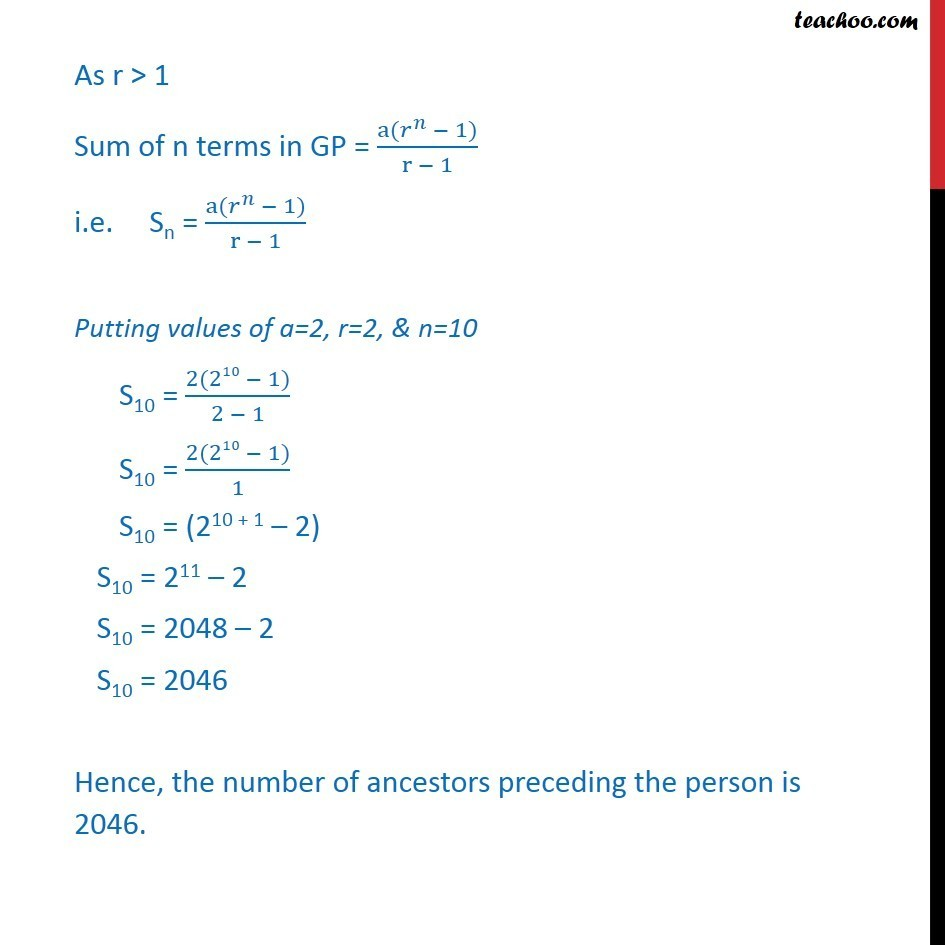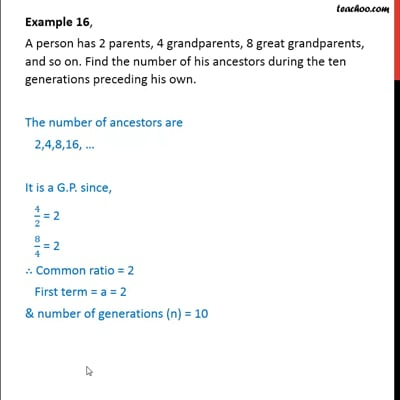Examples

Chapter 9 Class 11 Sequences and Series
Serial order wiseThis video is only available for Teachoo black users

Solve all your doubts with Teachoo Black (new monthly pack available now!)

### Transcript

Example 16, A person has 2 parents, 4 grandparents, 8 great grandparents, and so on. Find the number of his ancestors during the ten generations preceding his own. The number of ancestors are 2,4,8,16, … It is a G.P. since, 4/2 = 2 8/4 = 2 ∴ Common ratio = 2 First term = a = 2 & number of generations (n) = 10 We have to find the number of his ancestors during the ten generations preceding his own, i.e. S10 As r > 1 Sum of n terms in GP = (a(𝑟^𝑛 − 1))/(r − 1) i.e. Sn = (a(𝑟^𝑛 − 1))/(r − 1) Putting values of a=2, r=2, & n=10 S10 = (2(210 − 1))/(2 − 1) S10 = (2(210 − 1))/1 S10 = (210 + 1 – 2) S10 = 211 – 2 S10 = 2048 – 2 S10 = 2046 Hence, the number of ancestors preceding the person is 2046.